# 20. Contour Plots with Matplotlib

## Contour Plot

A contour line or isoline of a function of two variables is a curve along which the function has a constant value.

It is a cross-section of the three-dimensional graph of the function f(x, y) parallel to the x, y plane.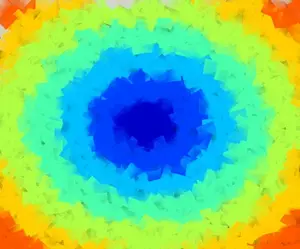Contour lines are used e.g. in geography and meteorology.

In cartography, a contour line joins points of equal elevation (height) above a given level, such as mean sea level.

We can also say in a more general way that a contour line of a function with two variables is a curve which connects points with the same values.

### Creating a "meshgrid"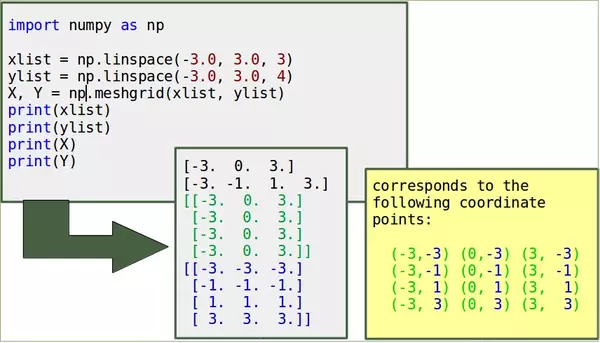# the following line is only necessary if working with "ipython notebook"
%matplotlib inline

import matplotlib.pyplot as plt
import numpy as np

n, m = 7, 7
start = -3

x_vals = np.arange(start, start+n, 1)
y_vals = np.arange(start, start+m, 1)
X, Y = np.meshgrid(x_vals, y_vals)

print(X)
print(Y)


### OUTPUT:

[[-3 -2 -1  0  1  2  3]
[-3 -2 -1  0  1  2  3]
[-3 -2 -1  0  1  2  3]
[-3 -2 -1  0  1  2  3]
[-3 -2 -1  0  1  2  3]
[-3 -2 -1  0  1  2  3]
[-3 -2 -1  0  1  2  3]]
[[-3 -3 -3 -3 -3 -3 -3]
[-2 -2 -2 -2 -2 -2 -2]
[-1 -1 -1 -1 -1 -1 -1]
[ 0  0  0  0  0  0  0]
[ 1  1  1  1  1  1  1]
[ 2  2  2  2  2  2  2]
[ 3  3  3  3  3  3  3]]


We can visulize our meshgridif we add the following code to our previous program:

fig, ax = plt.subplots()

ax.scatter(X, Y, color="green")
ax.set_title('Regular Grid, created by Meshgrid')
ax.set_xlabel('x')
ax.set_ylabel('y')
plt.show()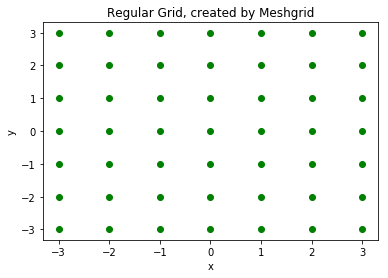import numpy as np

xlist = np.linspace(-3.0, 3.0, 3)
ylist = np.linspace(-3.0, 3.0, 4)
X, Y = np.meshgrid(xlist, ylist)

Z = np.sqrt(X**2 + Y**2)
print(Z)


### OUTPUT:

[[4.24264069 3.         4.24264069]
[3.16227766 1.         3.16227766]
[3.16227766 1.         3.16227766]
[4.24264069 3.         4.24264069]]


### Calculation of the Values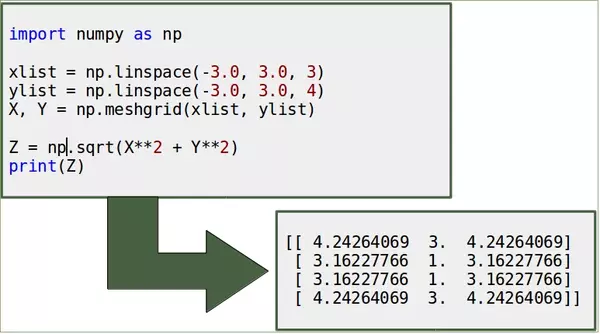import numpy as np

xlist = np.linspace(-3.0, 3.0, 3)
ylist = np.linspace(-3.0, 3.0, 4)
X, Y = np.meshgrid(xlist, ylist)

Z = np.sqrt(X**2 + Y**2)
print(Z)


### OUTPUT:

[[4.24264069 3.         4.24264069]
[3.16227766 1.         3.16227766]
[3.16227766 1.         3.16227766]
[4.24264069 3.         4.24264069]]

fig = plt.figure(figsize=(6,5))
left, bottom, width, height = 0.1, 0.1, 0.8, 0.8
ax = fig.add_axes([left, bottom, width, height])

Z = np.sqrt(X**2 + Y**2)
cp = ax.contour(X, Y, Z)
ax.clabel(cp, inline=True,
fontsize=10)
ax.set_title('Contour Plot')
ax.set_xlabel('x (cm)')
ax.set_ylabel('y (cm)')
plt.show()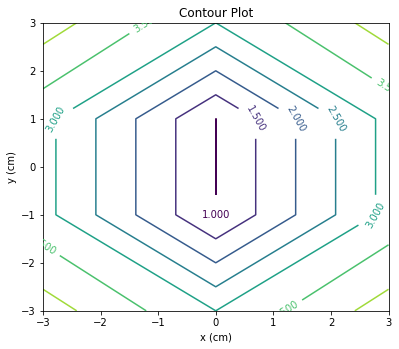### Changing the Colours and the Line Style

import matplotlib.pyplot as plt

plt.figure()
cp = plt.contour(X, Y, Z, colors='black', linestyles='dashed')
plt.clabel(cp, inline=True,
fontsize=10)
plt.title('Contour Plot')
plt.xlabel('x (cm)')
plt.ylabel('y (cm)')
plt.show()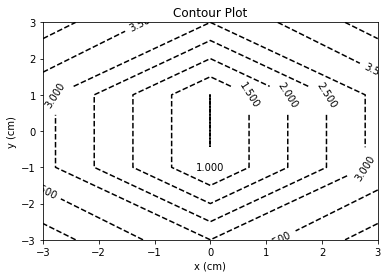### Filled Contours

import matplotlib.pyplot as plt
import numpy as np

fig = plt.figure(figsize=(6,5))
left, bottom, width, height = 0.1, 0.1, 0.8, 0.8
ax = fig.add_axes([left, bottom, width, height])

start, stop, n_values = -8, 8, 800

x_vals = np.linspace(start, stop, n_values)
y_vals = np.linspace(start, stop, n_values)
X, Y = np.meshgrid(x_vals, y_vals)

Z = np.sqrt(X**2 + Y**2)

cp = plt.contourf(X, Y, Z)
plt.colorbar(cp)

ax.set_title('Contour Plot')
ax.set_xlabel('x (cm)')
ax.set_ylabel('y (cm)')
plt.show()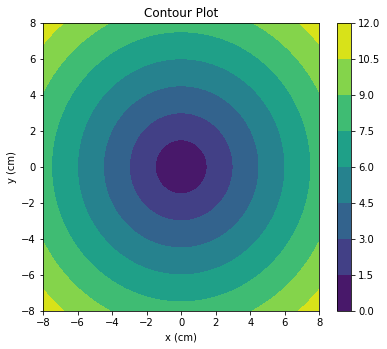### Individual Colours

import numpy as np
import matplotlib.pyplot as plt

xlist = np.linspace(-3.0, 3.0, 100)
ylist = np.linspace(-3.0, 3.0, 100)
X, Y = np.meshgrid(xlist, ylist)
Z = np.sqrt(X**2 + Y**2)

plt.figure()

contour = plt.contour(X, Y, Z)
plt.clabel(contour, colors = 'k', fmt = '%2.1f', fontsize=12)
c = ('#ff0000', '#ffff00', '#0000FF', '0.6', 'c', 'm')
contour_filled = plt.contourf(X, Y, Z, colors=c)
plt.colorbar(contour_filled)

plt.title('Filled Contours Plot')
plt.xlabel('x (cm)')
plt.ylabel('y (cm)')
plt.savefig('contourplot_own_colours.png', dpi=300)
plt.show()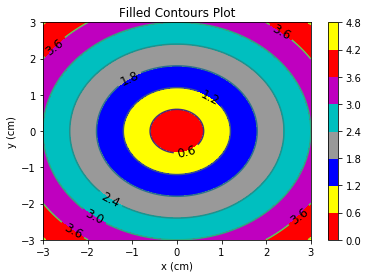### Levels

The levels were decided automatically by contour and contourf so far. They can be defined manually, by providing a list of levels as a fourth parameter. Contour lines will be drawn for each value in the list, if we use contour. For contourf, there will be filled colored regions between the values in the list.

import numpy as np
import matplotlib.pyplot as plt

xlist = np.linspace(-3.0, 3.0, 100)
ylist = np.linspace(-3.0, 3.0, 100)
X, Y = np.meshgrid(xlist, ylist)

Z = np.sqrt(X ** 2 + Y ** 2 )
plt.figure()

levels = [0.0, 0.2, 0.5, 0.9, 1.5, 2.5, 3.5]
contour = plt.contour(X, Y, Z, levels, colors='k')
plt.clabel(contour, colors = 'k', fmt = '%2.1f', fontsize=12)
contour_filled = plt.contourf(X, Y, Z, levels)
plt.colorbar(contour_filled)

plt.title('Plot from level list')
plt.xlabel('x (cm)')
plt.ylabel('y (cm)')
plt.show()


The last example of this chapter will be a "lovely" contour plot:

import matplotlib.pyplot as plt
import numpy as np

y, x = np.ogrid[-1:2:100j, -1:1:100j]
plt.contour(x.ravel(),
y.ravel(),
x**2 + (y-((x**2)**(1.0/3)))**2,
,
colors='red',)
plt.axis('equal')
plt.show()


Live Python training

Enjoying this page? We offer live Python training courses covering the content of this site.

Upcoming online Courses

Enrol here